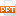# Bài giảng Database System - 5. Relational Algebra

Brief Introduction to Relational Calculus Another variation of relational calculus called the domain relational calculus, or simply, domain calculus is equivalent to tuple calculus and to relational algebra QBE (Query-By-Example): see Appendix D Domain calculus differs from tuple calculus in the type of variables used in formulas: rather than having variables range over tuples, the variables range over single values from domains of attributes. To form a relation of degree n for a query result, we must have n of these domain variables—one for each attribute An expression of the domain calculus is of the form {x1, x2, . . ., xn | COND(x1, x2, . . ., xn, xn+1, xn+2, . . ., xn+m)} where x1, x2, . . ., xn, xn+1, xn+2, . . ., xn+m are domain variables that range over domains (of attributes) and COND is a condition or formula of the domain relational calculus60 trang | Chia sẻ: vutrong32 | Ngày: 19/10/2018 | Lượt xem: 266 | Lượt tải: 0
Bạn đang xem trước 20 trang tài liệu Bài giảng Database System - 5. Relational Algebra, để xem tài liệu hoàn chỉnh bạn click vào nút DOWNLOAD ở trên
•database_systems_nguyenthanhtung_lec5_7684.ppt